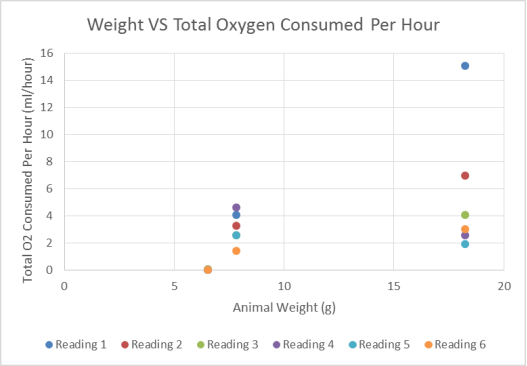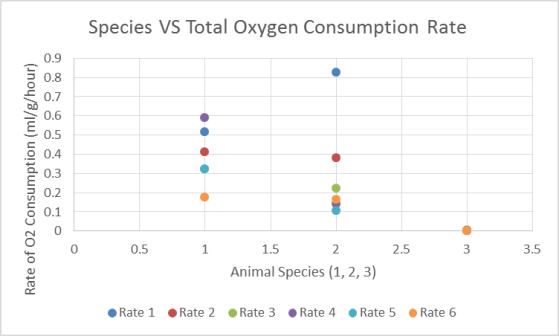HELLO, GUEST## Oxygen Consumption By Different Animals

Oxygen Consumption By Different Animals

 Name & Matric No. Calvin Belulok Ngang 40754 Malinka Anak Sikim 41989 Philovenny Anak Pengiran 43733 Brenda May Engkian anak Nandong @ Sulie 40747 Christ Hani binti Lian Lee 40907 Racheal Anak Rosedy 43768 Hilda Jelembai Anak Nelson Ilan 41423 Muazzah Bt Abd Latif 42327

LABORATORY 2

INTRODUCTION

There are two types of respiration namely the internal and external respiration. As mentioned by Rastogi (1982) the internal respiration or also known as cellular respiration involved the supply of oxygen to the tissues and the production of carbon dioxide and metabolites due to metabolism activities while external respiration is basically the mechanism effects of taking the air into the lungs. Anabolism and catabolism are under the metabolism processes. The metabolism processes in the animals body depends on the nutrient and oxygen supplies.

This experiment is to measure the respiration in animals by measuring the rate of oxygen consumption per gram mass per hour. The measurement of rate of oxygen consumption is to determine the metabolism rate of the animals (Rastogi, 1982). The physiological states of the animals affect the rate of oxygen consumption. Thus the animals used in this experiment must be mentally and physically at rest and the body activity must be reduced to minimum. The energy produced under these conditions represents the basal metabolism rate. (Siddiqi, 1982)

The comparison of lungs structures (we are using two different species of frogs) and the fish gills to observed the differences and understand the adaptations and advantages as well as disadvantages of these two structures.

METHODOLOGY

For the frogs :

The respirometer is set up as shown in the following diagram (figure 1). It consists of a wide-mouth bottle with an airtight-cork. Through the cork, a fine 1 ml graduated pipette is inserted. No leaks are ensured in the assembly (silicon rubber or grease is used to plug any leaks). The bottle is used as an animal chamber. A small platform made of wire mesh is made. Cotton is briefly soaked in 15% potassium hydroxide (KOH) solution and has put under the wire mesh so that it did not come into direct contact with the frogs. KOH is used to absorb the carbon dioxide produced by the frogs during respiration. In this way, the marker moved in one way, inward.

The frogs of relatively small size are put inside a plastic bag and weighed to the nearest mg and introduced into the chamber. The cork fitted with pipette is put and syringed and tightly fitted to the mouth of the bottle. The frogs are kept at rest for at least one minute inside the chamber before experiment started. A drop of coloured indicator solution (marker) is added in the pipette and adjusted to start position (0.0 ml) using the syringe. The movement of the marker is recorded at 5 minutes interval for 30 minutes. As the frogs started to consume oxygen, the indicator moved inwards. Temperature or pressure alteration affected the displacement of the marker. The experiment is repeated with a dead specimen (or something with the same mass as the specimen in place of the organism) to control these factors. The displacement of the second experiment from the first is calculated.

A Sultan fish is taken out from the bag and swiftly put into the ice to kill it. It was put on the aluminium paper for operation. Sultan fish is then operated by slicing it from mouth to stomach to take out the gills using small surgical blade. The gills are slowly pulled so that it was in a good condition. the gills are then wiped with tissue and observed carefully. The structure of gills is sketched and labelled.

RESULT AND CALCULATIONS

Total oxygen consumed per hour = d × v × (60/t) = x ml per hour

Rate of oxygen consumption = x/w ml/g/hour

Weight = 7.8495 g

Total oxygen consumed per hour = 0.58 cm × 0.58 ml/1 cm × (60/5) h-1

= 0.3364 ml × 12 h-1

= 4.0368 ml/hour

Rate of oxygen consumption = 4.0368 ml h-1/7.8495 g

= 0.5143 ml/g/hour

Total oxygen consumed per hour = 0.52 cm × 0.52 ml/1 cm × (60/5) h-1

= 0.2704 ml × 12 h-1

= 3.2448 ml/hour

Rate of oxygen consumption = 3.2448 ml h-1/7.8495 g

= 0.4134 ml/g/hour

Total oxygen consumed per hour = 0.46 cm × 0.46 ml/1 cm × (60/5) h-1

= 0.2116 ml × 12 h-1

= 2.5392 ml/hour

Rate of oxygen consumption = 2.5392 ml h-1/7.8495 g

= 0.3235 ml/g/hour

Total oxygen consumed per hour = 0.62 cm × 0.62 ml/1 cm × (60/5) h-1

= 0.3844 ml × 12 h-1

= 4.6128 ml/hour

Rate of oxygen consumption = 4.6128 ml h-1/7.8495 g

= 0.5877 ml/g/hour

Total oxygen consumed per hour = 0.46 cm × 0.46 ml/1 cm × (60/5) h-1

= 0.2116 ml × 12 h-1

= 2.5392 ml/hour

Rate of oxygen consumption = 2.5392 ml h-1/7.8495 g

= 0.3235 ml/g/hour

Total oxygen consumed per hour = 0.34 cm × 0.34 ml/1 cm × (60/5) h-1

= 0.1156 ml × 12 h-1

= 1.3872 ml/hour

Rate of oxygen consumption = 1.3872 ml h-1/7.8495 g

= 0.1767 ml/g/hour

Weight = 18.2584 g

Total oxygen consumed per hour = 1.12 cm × 1.12 ml/1 cm × (60/5) h-1

= 1.2544 ml × 12 h-1

= 15.0528 ml/hour

Rate of oxygen consumption = 15.0528 ml h-1/18.2584 g

= 0.8244 ml/g/hour

Total oxygen consumed per hour = 0.76 cm × 0.76 ml/1 cm × (60/5) h-1

= 0.5776 ml × 12 h-1

= 6.9312 ml/hour

Rate of oxygen consumption = 6.9312 ml h-1/18.2584 g

= 0.3796 ml/g/hour

Total oxygen consumed per hour = 0.58 cm × 0.58 ml/1 cm × (60/5) h-1

= 0.3364 ml × 12 h-1

= 4.0368 ml/hour

Rate of oxygen consumption = 4.0368 ml h-1/18.2584 g

= 0.2211 ml/g/hour

Total oxygen consumed per hour = 0.46 cm × 0.46 ml/1 cm × (60/5) h-1

= 0.2116 ml × 12 h-1

= 2.5392 ml/hour

Rate of oxygen consumption = 2.5392 ml h-1/18.2584 g

= 0.1391 ml/g/hour

Total oxygen consumed per hour = 0.40 cm × 0.40 ml/1 cm × (60/5) h-1

= 0.1600 ml × 12 h-1

= 1.9200 ml/hour

Rate of oxygen consumption = 1.9200 ml h-1/18.2584 g

= 0.1052 ml/g/hour

Total oxygen consumed per hour = 0.50 cm × 0.50 ml/1 cm × (60/5) h-1

= 0.2500 ml × 12 h-1

= 3.0000 ml/hour

Rate of oxygen consumption = 3.0000 ml h-1/18.2584 g

= 0.1643 ml/g/hour

Weight = 6.5485 g

Total oxygen consumed per hour = 0.03 cm × 0.03 ml/1 cm × (60/5) h-1

= 0.0009 ml × 12 h-1

= 0.0108 ml/hour

Rate of oxygen consumption = 0.0108 ml h-1/6.5485 g

= 0.0016 ml/g/hour

Total oxygen consumed per hour = 0.04 cm × 0.04 ml/1 cm × (60/5) h-1

= 0.0016 ml × 12 h-1

= 0.0192 ml/hour

Rate of oxygen consumption = 0.0192 ml h-1/6.5485 g

= 0.0029 ml/g/hour

Total oxygen consumed per hour = 0.05 cm × 0.05 ml/1 cm × (60/5) h-1

= 0.0025 ml × 12 h-1

= 0.0300 ml/hour

Rate of oxygen consumption = 0.0300 ml h-1/6.5485 g

= 0.0046 ml/g/hour

Total oxygen consumed per hour = 0.03 cm × 0.03 ml/1 cm × (60/5) h-1

= 0.0009 ml × 12 h-1

= 0.0108 ml/hour

Rate of oxygen consumption = 0.0108 ml h-1/6.5485 g

= 0.0016 ml/g/hour

Total oxygen consumed per hour = 0.01 cm × 0.01 ml/1 cm × (60/5) h-1

= 0.0001 ml × 12 h-1

= 0.0012 ml/hour

Rate of oxygen consumption = 0.0012 ml h-1/6.5485 g

= 0.0002 ml/g/hour

Total oxygen consumed per hour = 0.00 cm × 0.00 ml/1 cm × (60/5) h-1

= 0.0000 ml × 12 h-1

= 0.0000 ml/hour

Rate of oxygen consumption = 0.0000 ml h-1/6.5485 g

= 0.0000 ml/g/hour

Table 1: Weight VS Total Oxygen Consumed Per Hour

Graph 1: Weight VS Total Oxygen Consumed Per HourTable 2: Species VS Total Oxygen Consumption Rate

 Species Rate 1 Rate 2 Rate 3 Rate 4 Rate 5 Rate 6 Toad 1 0.5143 0.4134 0.3235 0.5877 0.3235 0.1767 Toad 2 0.8244 0.3796 0.2211 0.1391 0.1052 0.1643 Dead specimen 0.0016 0.0029 0.0046 0.0016 0.0002 0

Graph 2: Species VS Total Oxygen Consumption RateDISCUSSION

A graph of Weight Vs Total Oxygen Consumed is plotted based on the experimental results. The x-axis represents the weight of the animals, while the y-axis represents the rate of oxygen consumption. The weight of the animals gives effect towards the rate of oxygen consumption.

Another graph of Species Vs Total Oxygen Consumption Rate was plotted according to the experimental results. Different species shows different oxygen consumption rate. Different species have different surface area for oxygen absorption. Therefore the rate of oxygen consumption in each species differs.

Rate of respiration depends upon blood carbon dioxide level expressed as CO2, which in turn controls the series of reflexes to adjust changed respiratory demands of the body. Respiration is accelerated when the oxygen and carbon dioxide contents fluctuates. Respiration process itself depends on site of gaseous exchange. Oxygen concentration is greater in lung alveoli(101 mmHg) than in blood of the lung capillaries(40 mmHg). Oxygen will diffuses naturally into the blood capillaries. Carbon dioxide diffuses from blood into the alveoli thus diffusion of both oxygen and carbon dioxide gases, there is diffusion gradient that determines the direction of their transport.

The oxygen consumption measurements were made on two animals of different body masses which are 7.8496g and 18.2584g. The bigger the body size of an animal, the higher the body mass, the higher the total oxygen consumption for the animal. However, specific oxygen consumption which is the rate of O2 consumption per unit body mass will decreased with the increased of body mass and size. As the body size and mass increases, the metabolic rate also increases.

The rate of oxygen consumption is measured as the rate of oxidative metabolism. Not all animals have similar rates of oxygen consumed. This is because they are influenced by numerous factors. For example, their environment, physiology, non-locomotory, activity metabolism, body mass and size. Small animals have less total oxygen consumption than the large ones. For animals that have similar physiology, their rates of oxygen consumption increases as their body mass increases. Toad is a poikilothermic. Their body temperature is near to the ambient temperature. The metabolic rate of amphibians and fish are sensitive to temperature and other variables which also shows a strong requirement on the oxygen supply. It is stated (Fox & Wingfield, 1937) that when two species within one genus are compared, the relation of oxygen consumption to temperature can be stated by a single curve. Species that live at higher temperatures consume more oxygen that arctic species of same genus that live in lower temperatures. Therefore, the rate of oxygen consumption of animals from warm and colder habitats is considered.

CONCLUSION

The rate of oxygen consumption to determine the metabolism rate of the animals is measured considering the physiological states of the animals. The comparison of lungs structures and fish gills by observing the advantages and disadvantages of each structures of the organ.## Most Used Categories

Testimonials
I order from this writer for quite a while, so we are having the chemistry going on between us. Great job as always!
Laura C., March 2018
Wow, ordering from EssayHub was one of the most pleasant experiences I have ever had. Not only was my work sent to me hours before the deadline, but the content was absolutely fantastic! Would order from them again!
Daniel L., March 2018
Professional Custom
Professional Custom Essay Writing Services
In need of qualified essay help online or professional assistance with your research paper?
Browsing the web for a reliable custom writing service to give you a hand with college assignment?
Out of time and require quick and moreover effective support with your term paper or dissertation?